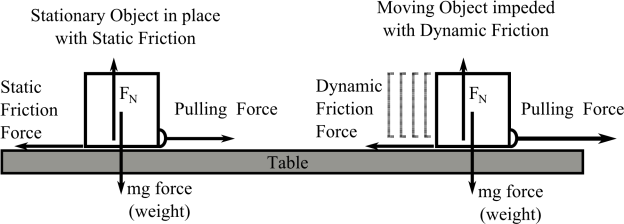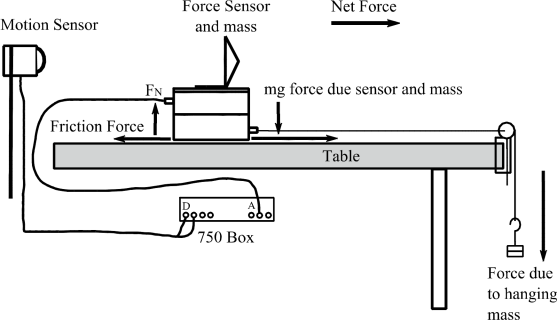Disclaimer: This is an example of a student written essay.

Any scientific information contained within this essay should not be treated as fact, this content is to be used for educational purposes only and may contain factual inaccuracies or be out of date.

# Friction Experiment: Design and Results

 ✅ Paper Type: Free Essay ✅ Subject: Physics ✅ Wordcount: 1937 words ✅ Published: 9th Mar 2018

EXPERIMENT 37

Friction

## I. Introduction

Whatever action you do whether it is walking, driving, or when any two surfaces meet there is friction between them. Friction opposes the applied force to an object and opposes the motion of an object. In many of the labs in this course we try to minimize it or neglect it in the lab, but it is there. When we use the air track, the friction is dramatically reduced due to the air cushion under the air car so the car stays in motion for an extended period of time, but it still stops. Or in the case of an oscillating object, we ignore the slowing of the oscillation, but it still slows down and stops. The actual cause of friction is complex atomic interaction but, the simple idea of friction is atoms rubbing against each other, adsorbing energy from the motion.

If you need assistance with writing your essay, our professional essay writing service is here to help!

Friction is a force; it prevents an object from moving or changes the motion of an object. This lab will cover two types of friction, static friction and kinetic friction. Static friction is a force that resists motion so that the surfaces are not in motion relative to each other. The maximum amount of force applied to the block, at the instant before the block moves, is referred to as the maximum static friction force, f S Max. One example of this type of friction is walking.

Once enough force is applied to the system to overcome the static friction force, it starts to move. When the block is moving against the surface, then the friction force is called the kinetic friction force, f k. Kinetic friction appears when the two surfaces are sliding relative to each other. One example of this type of friction is pushing a file cabinet across the floor.

In this lab you will pull a weighted block across the table and measure the force it takes to start moving the block (just an instant before it moves) and while the block is moving across the table. The forces in this lab are many, the block exerts a force on the table, the table exerts a force on the block ( fN ). And the earth exerts a force on the block (mg) and the block exerts a force on the earth. This experiment will consider the room and table as stationary objects and therefore having no accelerating force on them, then the net force on the stationary block is

fnet = 0 (1).

The force of the block on the table is equal to the force of the block on the earth, weight or mg,

mg – fN = fNet (2) thus

mg = fN (3).Figure 1: Diagram of two blocks one stationary and one moving. The stationary object is held back by static fraction, while the moving object is acted on by friction and a pulling force.

The static friction force acts equal and opposite to the pulling force, as the pulling force increases the static friction force increases, resulting in no motion. Sometimes the pulling force will increases and it will exceed the static friction and the block will begin to move. The point of maximum force is called maximum static force, f SMax. An observation about static friction is that maximum static friction f SMax is proportional to the normal force, fN, through a constant µs, f SMax = µs fN. (4)

The µs term is referred to as the coefficient of static friction. This means as the normal force ( fN ) increases, the maximum force needed to move the block increases in a proportional amount. The coefficient of static friction is dependent on the two surfaces in contact so different surfaces will have different coefficients of friction. A second observation about friction is that friction is independent of the size of the contact area between the two solid surfaces, which means the same force spread over different areas still would have the same force of friction.

Kinetic friction like static friction is a retarding force exerted on a sliding object in contact with a surface. When the object is sliding with a constant velocity the force of friction is equal to the pulling force. It follows the same equation as static friction but the relationship between kinetic friction and the normal force has a different coefficient. The coefficient is referred to as the kinetic coefficient of friction µk.

## fk = µkfN. (5)

Kinetic friction also does not change when the surface area of the two surfaces changes. You will be measuring both static and kinetic friction forces in this lab and you should find that the kinetic friction is usually lower that the maximum static friction.

## II. Equipment and Procedure

IIa. Equipment: Force sensor, block, motion sensor, laptop PC, 750 interface, friction surface aka table, string, pulley, weights and weight hanger.Figure 2: Equipment setup of the friction experiment.

The hanging mass will pull the force sensor with a mass, while the motion sensor will measure the displacement of the force sensor. Once the hanging mass force exceeds the friction force, the force sensor will move, and the motion sensor will measure the displacement. The moving force sensor will have a velocity measured by the computer, and the net force on the force sensor will be measured.

IIb. Procedure:

The mass of the block and force sensor needs to be measured so that the total mass of the block/force sensor on the table can be determined.

Hook the motion sensor and the force sensor to the 750 interface box and hook the interface box to the laptop. The force sensor is measuring the force exerted on the block while the motion sensor will measure the change in distance of the block. Turn on the computer and 750 interface, start the Data Studio program and create an experiment. Select a digital port and add the motion sensor to the experiment. Double click on the motion sensor to open the settings of the motion sensor, set the frequency rate to 25 Hz and close the window. Drag the motion sensor icon in the upper left to the graph icon in the lower left. Go to an analog port on the 750 interface box and add the force sensor to the experiment, double click on the force sensor to open the sensor settings, set the frequency to a minimum of 500 Hz. Drag the force sensor icon in the upper left to the lower left graph icon. One reminder is to hit the tare button every time before you run an experiment. This action resets the force sensor to zero Newtons before each run.

Static Friction Experiment: part one

1. Start the experiment, tare the force sensor.
2. Add the hanger and add weight incrementally. As you try more runs use smaller masses for your increment.
3. Keep adding weight until the block starts to move.
4. Once the mass moves, stop the experiment.
5. Repeat the experiment 6 times to get an average value and perform standard deviation (SD) on your values.

Kinetic Friction Experiment: part two

1. Start the experiment, tare the force sensor.
2. Pull the force sensor using the string to make the block move.
3. Once the block is moving at a constant velocity, this will indicate what force is needed to match the kinetic friction.
4. Plot the displacement vs. time from the motion sensor. Fit the curve to a linear function to show that the block has a uniform velocity.
5. Repeat the experiment 6 times to get an average value and perform SD error analysis.

Kinetic Friction Experiment: part three

1. Start the experiment and tare the force sensor.
2. Add the mass required to move the block with 100 grams extra.
3. The block will start to move with an accelerating velocity, if not add an extra 50 grams until it does. The plot of the position vs. time will determine if the block is accelerating.

Question: What should the plot look like if the block is accelerating?

1. Once a run is complete with the block accelerating along the table, stop the experiment.
2. Plot the displacement vs. time from the motion sensor. Fit the curve to a quadratic function to find the acceleration of the block.
3. Repeat the experiment 6 times to get an average value and perform SD error analysis.

## III. Data

The graph of the force vs. time or determines the maximum value of the force. The maximum force is the static friction force.

In part two (kinetic friction), drag the block at a uniform velocity. The plot of time vs. displacement will clearly identify the linear motion. Use a linear formula to fit the curve if necessary. Measure the force on the block when it is moving.

In part three (kinetic friction), drag the block with an accelerating force and generate a plot time vs. displacement in a graph. Fit the curve to a quadratic formula and determine the acceleration of the block. The acceleration of the block is used to determine the net force on the block. The net force on the block is the difference between the force of the mass hanging down and the force of friction holding it back. One reminder is the displacement of a moving object is related to the acceleration through equation (6).

=(6)

## IV. Results

Calculate the coefficient of static friction of the block, from the force exerted on the block and the mass and force of the block on the table. Calculate the SD from the values obtained in the experiment.

Calculate the kinetic friction force from the two different methods. First: calculate the kinetic friction from the constant velocity of the moving block. The force need to move the block at a constant velocity is equal to the kinetic friction force. Calculate the SD from the values obtained in the experiment. Second: calculate the kinetic friction difference from the accelerating block from the hanging force and the resultant force on the block. The mass of the block is known and the acceleration of the block is measured from the curve fit. The net force on the block can then be determined. The hanging force is known from mass times gravity (mg) and from that the force of kinetic friction can be calculated.

## V. Discussion

• What are values of the static and kinetic friction?
• Are the two values of kinetic friction similar? Are the kinetic friction values within the standard deviation?
• What happens when a sliding object has the pulling force reduced below the kinetic fiction force? How much force will it take to get it moving again?
• Is there a there large distribution in the values of static and kinetic friction? If so why?
• What would happen to the value of friction if the mass of the block is doubled?
• Is a wheel rolling, static or kinetic friction?
• Why can’t static friction be less that kinetic friction? Discuss the implication of this.

View all

## DMCA / Removal Request

If you are the original writer of this essay and no longer wish to have your work published on UKEssays.com then please:

Related Services

Prices from

BHD57

Approximate costs for:

• 1000 words
• 7 day deliveryHumanity University

Dedicated to your worth and value as a human being!

Related Lectures